×#### Thank you for registering.

One of our academic counsellors will contact you within 1 working day.

Click to Chat

1800-1023-196

+91-120-4616500

CART 0

• 0

MY CART (5)

Use Coupon: CART20 and get 20% off on all online Study Material

ITEM
DETAILS
MRP
DISCOUNT
FINAL PRICE
Total Price: Rs.

There are no items in this cart.
Continue Shopping• Complete JEE Main/Advanced Course and Test Series
• OFFERED PRICE: Rs. 15,900
• View Details

```Chapter 1: Integers Exercise – 1.4

Question: 1

Simplify

3 - (5 – 6 ÷ 3)

Solution:

3 - (5 – 6 ÷ 3)

= 3 – (5 – 2)

= 3 – 3

= 0

∴ 3 - (5 – 6 ÷ 3) = 0

Question: 2

Simplify

– 25 + 14 ÷ (5 - 3)

Solution:

-25 + 14 ÷ (5 - 3) = - 25 + 14 ÷ (2)

= - 25 + 14/2

= –25 + 7

= –18

∴ - 25 + 14 ÷ (5 - 3) = - 18

Question: 3

SimplifySolution: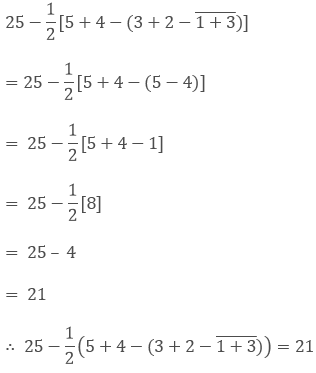Question: 4

Simplify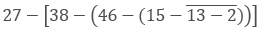Solution:Question: 5

Simplify

36 - [18 - (14 - (15 – 4 ÷ 2 × 2))]

Solution:

36 - [18 - (14 - (15 – 4 ÷ 2 × 2))]

= 36 - [18 - (14 - (11 ÷ 2 × 2))]

= 36 - [18 - (14 - 11/2 × 2))]

= 36 – [18 – (14 – 11)]

= 36 – [18 – 3]

= 36 – 15

= 21

∴ 36 – [18 – (14 – (15 – 4 ÷ 2 × 2))] = 21

Question: 6

Simplify

45 - [38 - (60 ÷ 3 - (6 – 9 ÷ 3) ÷ 3)]

Solution:

45 - [38 - (60 ÷ 3 - (6 – 9 ÷ 3) ÷ 3)]

= 45 - [38 - (20 - (6 - 3) ÷ 3)]

= 45 - [38 - (20 – 3 ÷ 3)]

= 45 – [38 – (20 – 1)]

= 45 – [38 – 19]

= 45 – 

= 26

∴ 45 - [38 - (60 ÷ 3 - (6 – 9 ÷ 3) ÷ 3)] = 26

Question: 7

Simplify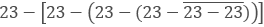Solution:= 23 – [23 – (23 – (23 – 0))]

= 23 – [23 – (23 – 23)]

= 23 – [23 – 0]

= 23 – 23

= 0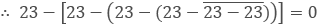Question: 8

Simplify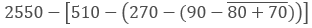Solution:= 2550 – [510 – (270 – (90 – 150))]

= 2550 – [510 – (270 – (–60))]

= 2550 – [510 – 330]

= 2550 – 

= 2550 – 180

= 2370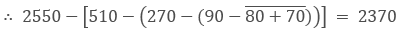Question: 9

Simplify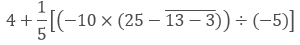Solution: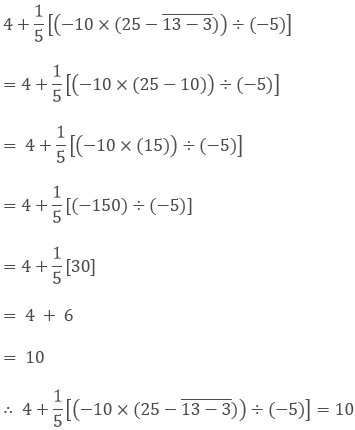Question: 10

SimplifySolution: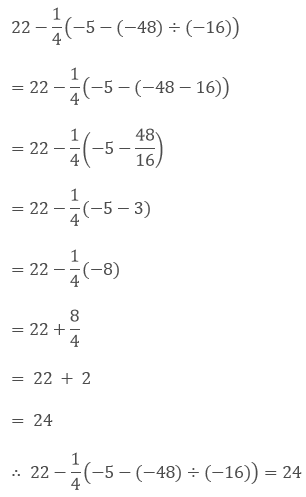Question: 11

SimplifySolution: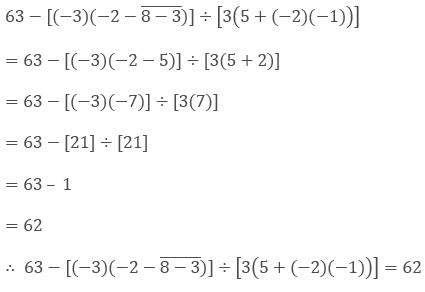Question: 12

Simplify

[29 - ( - 2)(6 - (7 - 3))] ÷ [3 × (5 + ( - 3) × (-2))]

Solution:

[29 - (-2)(6 - (7 - 3))] ÷ [3 × (5 + (- 3) × (- 2))]

= [29 - (- 2)(6 - 4)] ÷ [3 × (5 + (3 × 2))]

= [29 - (-2)(2)] ÷ [3 × (5 + 6)]

= [29 + 4] ÷ [3 × 11]

=  ÷ 

= 1

∴ [29 - (-2) (6 -(7- 3))] ÷ [3 × (5 + (- 3) × (-2))] = 1

Question: 13

Using brackets, write a mathematical expression for each of the following:

(i) Nine multiplied by the sum of two and five.

(ii) Twelve divided by the sum of one and three.

(iii) Twenty divided by the difference of seven and two.

(iv) Eight subtracted from the product of two and three.

(v) Forty divided by one more than the sum of nine and ten.

(vi) Two multiplied by one less than the difference of nineteen and six.

Solution:

(i) 9 (2 + 5)

(ii) 12 ÷ (1 + 3)

(iii) 20 ÷ (7 - 2)

(iv) 2 × 3 - 8

(v) 40 ÷ [1 + (9 + 10)]

(vi) 2 × [(19 - 6) - 1]
```### Course Features

• 728 Video Lectures
• Revision Notes
• Previous Year Papers
• Mind Map
• Study Planner
• NCERT Solutions
• Discussion Forum
• Test paper with Video Solution